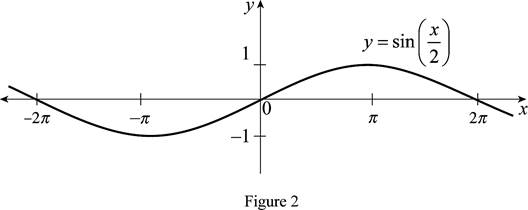# The function y = sin x by hand without plotting any points and then graph of the function y = sin ( 1 2 x ) .### Single Variable Calculus: Concepts...

4th Edition
James Stewart
Publisher: Cengage Learning
ISBN: 9781337687805### Single Variable Calculus: Concepts...

4th Edition
James Stewart
Publisher: Cengage Learning
ISBN: 9781337687805

#### Solutions

Chapter 1.3, Problem 19E
To determine

## To sketch: The function y=sinx by hand without plotting any points and then graph of the function y=sin(12x) .

Expert Solution

### Explanation of Solution

The standard graph of the function y=sinx is roughly drawn as shown below in Figure 1.Then, draw the graph of y=sin(12x) , stretch the graph y=sinx horizontally by a factor of 2. Thus, the graph of y=sin(12x) is shown below in Figure 2.Observe that Figure 2is obtainedfrom Figure 1 in such a way that it is stretchedhorizontally by a factor of 2.

### Have a homework question?

Subscribe to bartleby learn! Ask subject matter experts 30 homework questions each month. Plus, you’ll have access to millions of step-by-step textbook answers!